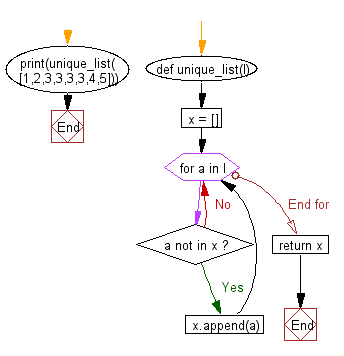﻿ Python Exercise: Unique elements from a list - w3resource# Python Exercise: Unique elements from a list

## Python Functions: Exercise-8 with Solution

Write a Python function that takes a list and returns a new list with unique elements of the first list.

Sample Solution:-

Python Code:

``````def unique_list(l):
x = []
for a in l:
if a not in x:
x.append(a)
return x

print(unique_list([1,2,3,3,3,3,4,5]))
```
```

Sample Output:

```[1, 2, 3, 4, 5]
```

Pictorial presentation:Flowchart:## Visualize Python code execution:

The following tool visualize what the computer is doing step-by-step as it executes the said program:

Python Code Editor:

Have another way to solve this solution? Contribute your code (and comments) through Disqus.

What is the difficulty level of this exercise?

Test your Programming skills with w3resource's quiz.

﻿

## Python: Tips of the Day

Decapitalizes the first letter of a string:

Example:

```def tips_decapitalize(s, upper_rest=False):
return s[:1].lower() + (s[1:].upper() if upper_rest else s[1:])
print(tips_decapitalize('PythonTips'))
print(tips_decapitalize('PythonTips', True))
```

Output:

```pythonTips
pYTHONTIPS
```